# How to solve a Rubik's cube 6x6 - Step 1

Before you assemble the Rubik's Cube 6x6, learn how to assemble the classic 3x3 cube, since the 6x6 cube assembly consists of the same algorithms as the 3x3, but supplemented by several new formulas

Step 1.

As with the other cubes, you must first turn it into a cube similar to the 3x3 cube. And then perform the classic cube formulas you already know.

At the beginning of this stage the cube is completely disassembled.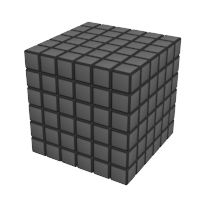At the end of the stage You should have all the middle pieces (16 cubes of one color).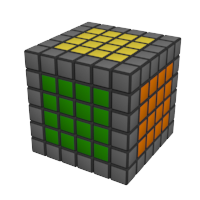The first thing to consider is that in a 6x6 cube the middles are not fixed as in a 3x3 cube, and it is important to place the colors of the middles correctly. They are placed the same way on almost all cubes.In order to perform this step, You need to rearrange the middle cubes between each other. Put the cubes You want in front of each other, and do the following formula.
If there is no suitable formula, rotate the sides of the cube so that you can use one of the formulas.

Variant 1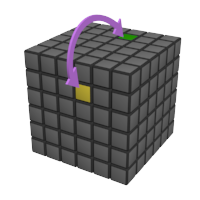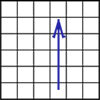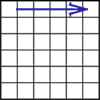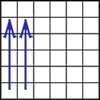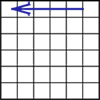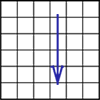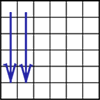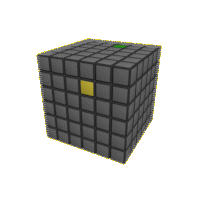Variant 2 (symmetrical)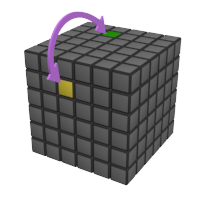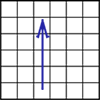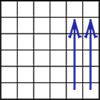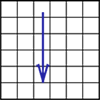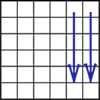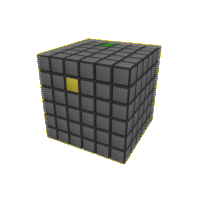Variant 3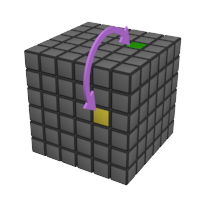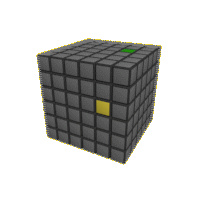Variant 4 (symmetrical)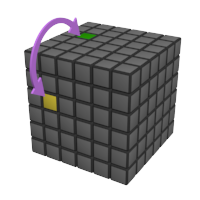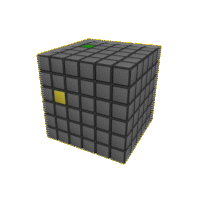Variant 5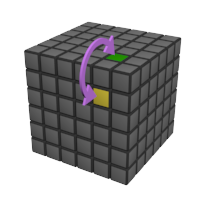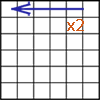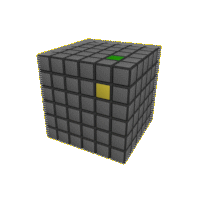Variant 6 (symmetrical)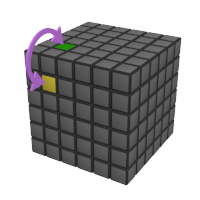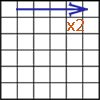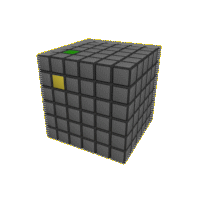Variant 7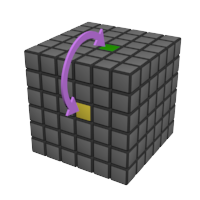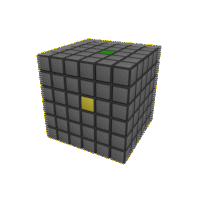Variant 8 (symmetrical)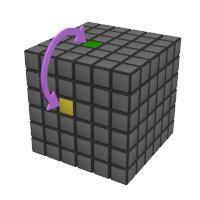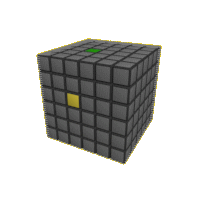Variant 9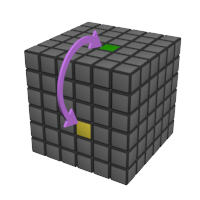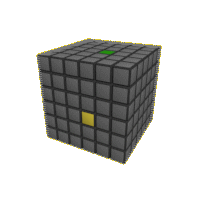Variant 10 (symmetrical)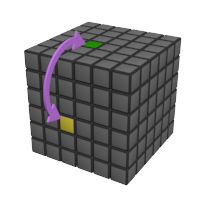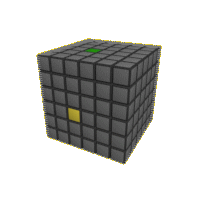If you decide to learn these formulas, don't learn the symmetric variants. First, you can do it without them, and second, if you learn the remaining formulas, you can reproduce the symmetric ones without memorizing them.
These formulas are easy to remember. Note that they all begin by turning up the line on which the desired dice are cubes. In this case, if the dice are on the 2nd or 5th lane, then two lines are turned (together with the 1st or 6th), and if the 3rd or 4th lane, then one.

* I'm sorry for my English. It's not my native language. If you want to help me with the correct construction of sentences and a selection of words, you can send me your version of the English text to my E-mail [email protected]